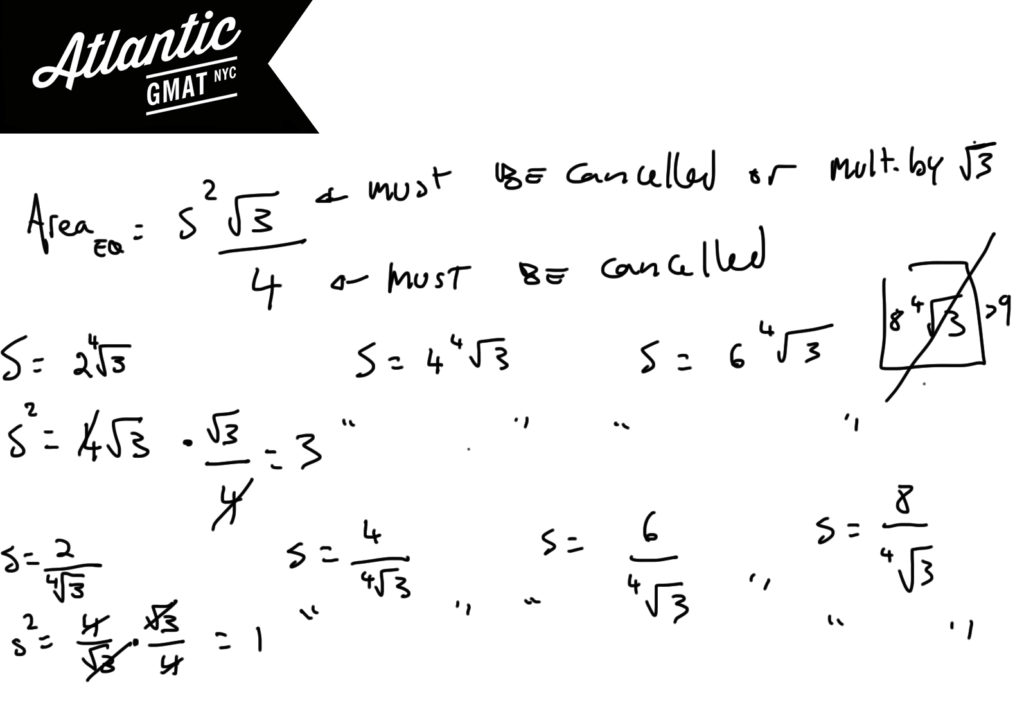# GMAT Question of the Day - Problem Solving - Geometry

If s is between 0 and 9, how many different equilateral triangles with side s can be formed that have an area which is an integer value?

A. 3

B. 4

C. 5

D. 6

E. 7

## GMAT Question of the Day Solution

This GMAT question of the day is tough but not impossible. What makes it tough? You have to consider a few different things while keeping in mind the limitations imposed by the question. First off - what's the area of an equilateral triangle? Now consider what numbers will create an integer value for the area? We have to get rid of the 4 in the denominator and the √3 in the numerator. Let's look at one issue at a time:

1. To cancel the 4 you need to have a at least a 4 in the numerator. It doesn't need to be a 4 but could be an 8 or a 12 or a 16. Anything with at least one 4 as a factor. Keep in mind the limitation that S must be less than 9. So that leaves 2, 4, 6, and 8 each of which when squared will have at least one 4.

2. We also need to either cancel or transform the √3. We can do this by either dividing or multiplying by √3. This means that S must have 3^1/4 in the numerator or the denominator.

Putting both together you have these options for S:

2(3^1/4), 4(3^1/4), 6(3^1/4)

2/(3^1/4), 4/(3^1/4), 6/(3^1/4), 8/(3^1/4)

Note that 8(3^1/4) will not work because it is greater than 9. That leaves seven different triangles.Sorry, comments are closed for this post.

# CONTACT

Atlantic GMAT

405 East 51st St.

NY, NY 10022

(347) 669-3545

info@AtlanticGMAT.com